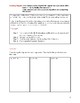# Solving quadratic equations by completing the squareSubject
Resource Type
File Type

PDF

(3 MB|34 pages)
Product Rating
Standards
• Product Description
• StandardsNEW

Learning target: I can analyze and solve quadratic equations by completing the square.

Before completing the square, you should teach completing the square for expression of the form x^2 + bx first. I have a free version of said lesson. Additionally, you should also teach solving quadratic equations using square roots (I've a paid version for this lesson) before completing the square. In this lesson, I included a brief lesson/practice on completing the square for expression of the form x^2 + bx and solving quadratic equations using square roots.

Teacher will model the example(s). Students will then use think-pair-share to answer the group-work problems. Students will present their work to the class.

Contact me if you need the workout solutions.

What's included:

- Do now

- Teacher modeling (when a = 1 and when a is ≠ to 1)

- Group-work (when a = 1 and when a is ≠ to 1)

Differentiation: you can have students do think pair share # 1; students will choose

any two of the letters to complete. You can have students do think pair share # 4;
they will choose between a or b. Also, you can have students do think pair share #
9; students will choose between a or b and then choose between c or d.

- Sample regents problems

- Error analysis

- Exit ticket (students have options)

- Homework

Use the method of completing the square to transform any quadratic equation in 𝘹 into an equation of the form (𝘹 – 𝘱)² = 𝘲 that has the same solutions. Derive the quadratic formula from this form.
Total Pages
34 pages
Included
Teaching Duration
N/A
Report this Resource to TpT
Reported resources will be reviewed by our team. Report this resource to let us know if this resource violates TpT’s content guidelines.